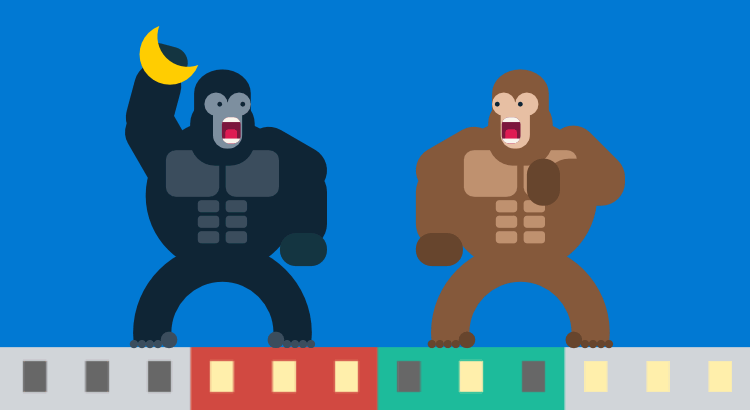# Supervised Learning Tutorial: AI Learns To Play Gorillas Game

In this supervised learning tutorial, I’m going to show my project in which AI learns to play Gorillas game using supervised machine learning algorithms.

To predict the outputs, this AI bot uses a simple neural network with 3 inputs, 16 hidden nodes and 2 outputs. The network is trained by applying polynomial regression to a bunch of data, previously collected by the brute-force method.

The game is programmed in Javascript using Phaser 2 framework.

To implement supervised machine learning algorithms I used Tensorflow JS library.

You can run and test the full simulation at the end of this machine learning game tutorial.

## 1. Video

Here is the video where I explain the entire project if you’d like to take a look at it:

## 2. Project Description

Let’s get started with a quick preview of this tensorflow js project.

As we know, the main goal was to make an AI bot that plays classic Gorillas game. Here I used artificial neural network in machine learning to predict shooting parameters. But at first, I had to trained it!

As in any other supervised machine learning example, the neural network learns to play by using relevant data. So, I needed to collect a bunch of data. Here I implemented a brute-force method to generate banana trajectories for a number of randomly created levels.

Finally, to fit the network, I applied polynomial regression to the collected data.

One more thing before we move on with this supervised learning example. In order to make a challenging project, I also defined the following goal:

• The AI bot must score more than 80 out of 100 shots!

## 3. Making Original Game

The first step of this project was to make the original fully playable Gorillas game, with complete functionality.

That means, I had to implement the original mechanics where two players throw explosive bananas trying to hit each other. To launch banana, they adjust the angle and power of each shot by using mouse.

Here you can play the original Gorillas game in your browser.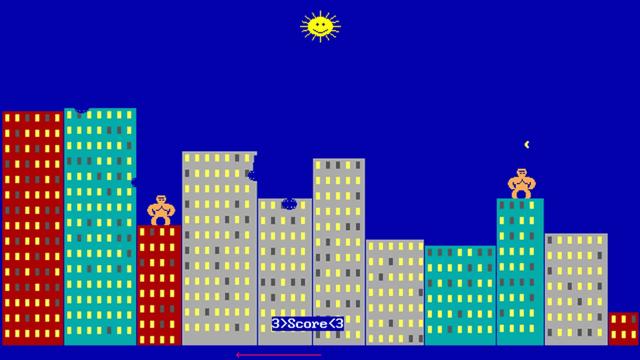## 4. Creating Neural Network Model

After completing the original game, the next step was to change it to AI learning game.

That means, I had to replace player controls with a neural network model.

So, instead of using mouse to define the shooting parameters, now the neural network would predict these values using supervised machine learning algorithms.

Here I used a simple neural network architecture consisted of 3 layers:

• an input layer with 3 nodes: view angle of the 1st gorilla, view angle of the 2nd gorilla, and the distance between both gorillas.
• a hidden layer with 16 nodes
• an output layer with 2 nodes: the power, and the angle of the shot.

The image below depicts this artificial neural network example: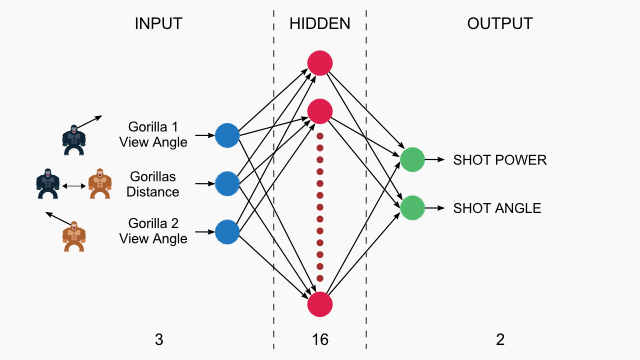## 5. Collecting Data

As I said before, to train supervised machine learning neural networks, we need data!

So, I implemented a brute force method to get shooting parameters for a number of random building configurations.

This method generates all possible banana trajectories for each random configuration. Then, it selects the middle trajectory as the best one to store it in the dataset.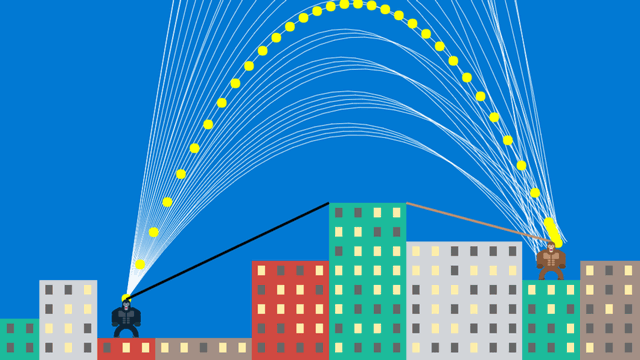## 6. Training with Polynomial Regression

Once I collected enough data, I was able to apply a non-linear regression (polynomial regression) to them to fit the neural network.

Here I used a polynomial model very similar to this common polynomial regression model example:
Y = A*(X^3) + B*(X^2) + C*(X) + D

The figure below illustrates the principle of polynomial regression: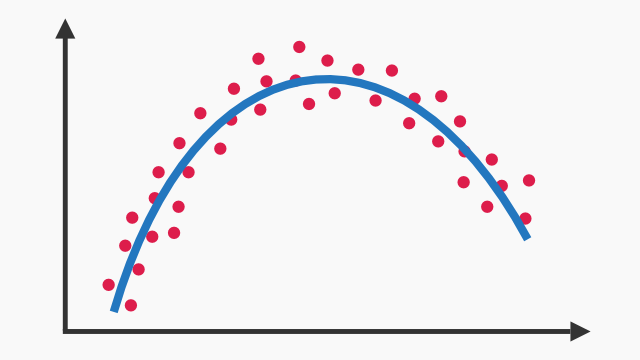## 7. Running Simulation

To conclude this supervised learning tutorial, let’s run the simulation!

To start a new game, press the “New Game” button. This opens the Options dialog where you can select:

1. dataset with [0 / 25k / 50k / 75k / 100k] data records
2. a pre-trained model to be loaded [yes / no]
3. ape size for the left player
4. ape size for the right player
5. maximal height difference between the buildings

After selecting all options, run the simulation by clicking on the “Play” button.

Now you can watch the fight between two AI bots.

The black line shows the view angle of the black ape (1. input), and the brown line shows the view angle of the brown ape (2. input). The distance between the gorillas is the 3. input.

To collect more data or train the model, press the appropriate buttons.

Please note that I have disabled all saving actions in the online version! So for instance, you can’t save your collected data or your trained model. Instead, this should be possible only if you download the prototype version from Github and run it locally on your computer.

## 8. Source Code

I hope you found this tensorflow js project interesting.

The source code of the prototype is available on Github:

https://github.com/ssusnic/Machine-Learning-Gorillas